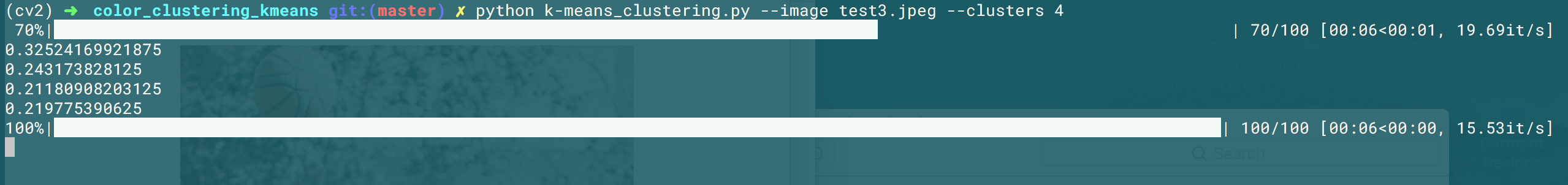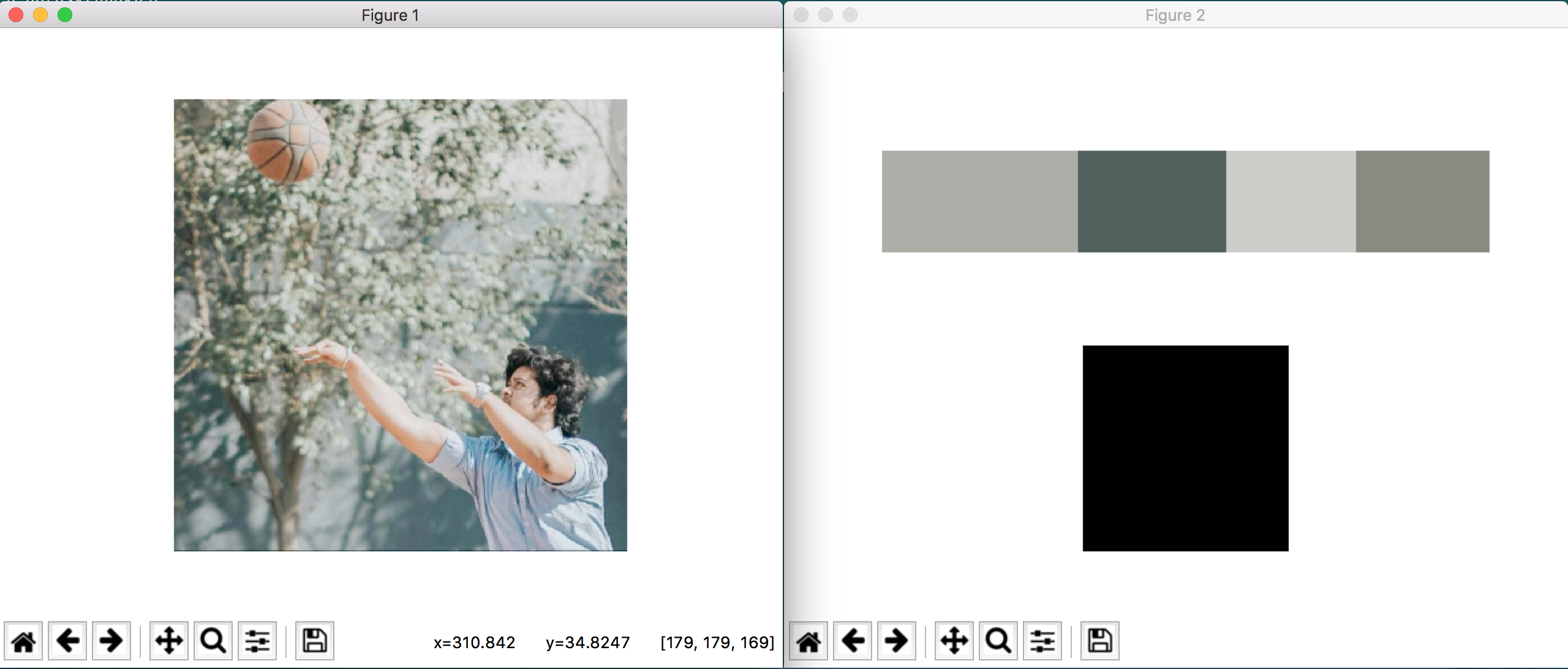# Blog post

06 June, 2020

## Color Clustering in Python

Educational blogTake a look at the MARVEL logo below. you can probably figure out the most dominant colors in a second. We clearly see Black, Red & WhiteIt's pretty easy for a human mind to pick these out. But what if we wanted to write an automated script that will take out the dominant colors from any image? Sounds cool right?

So that's what we're going to do today.

We will be using an algorithm known as the K-Means algorithm to automatically detect the dominant colors in the image.

### What is K-Means algorithm?

Our goal is to partition N data points into K clusters. Each of the N data points will be assigned to a cluster with the nearest mean. The mean of each cluster is called its centroid or center.

Applying k-means will yield K separate clusters of the original n data points. Data points inside a particular cluster are considered to be more similar to each other than data points that belong to other clusters.

In our case, we will be clustering the pixel intensities of an RGB image. Given an MxN size image, we thus have MxN pixels, each consisting of three components: Red, Green, and Blue respectively.

We will treat these MxN pixels as our data points and cluster them using k-means.

Pixels that belong to a given cluster will be more similar in color than pixels belonging to a separate cluster.

The only facet we need to keep in mind is that we will need to provide the number of clusters, K ahead of time

### The code

I'll try and explain the steps as we go through

``````from sklearn.cluster import KMeans
import matplotlib.pyplot as plot
import argparse
import cv2
import helper
from tqdm import tqdm
``````

We have imported all the dependencies needed for our project today

``````########################
pbar = tqdm(total = 100)
########################
``````
``````      0%|          | 0/100 [00:00<?, ?it/s][A
``````

This is just the run timer so that we can check on the efficiency at the end. As you can see, it shows 0% as of now

``````ap = argparse.ArgumentParser()
ap.add_argument("-i", "--image", required = True, default = "/Users/Interface/Coding 2/CV/color_clustering_kmeans/test3.jpeg")
ap.add_argument("-c", "--clusters", required = True, type = int, default = 4)
args = vars(ap.parse_args())
``````

We have added the CLI(command line) argument parser. Now we can send the arguments directly to the client while running the script.

``````# image = cv2.imread(args["image"])
image = cv2.cvtColor(image, cv2.COLOR_BGR2RGB)

########################
pbar.update(10)
########################

plot.figure()
plot.axis("off")
plot.imshow(image)

image = image.reshape(image.shape * image.shape, 3);
``````
``````     30%|â–ˆâ–ˆâ–ˆ       | 30/100 [02:27<05:21,  4.59s/it]
``````Here, we have fetched the image and converted that to an RGB color channel. An important point to note here is that openCV and most other image processing or Computer Vision frameworks take images in the BGR channel and not RGBNow, an image is basically an MxN matrix of pixels. We are simply re-shaping our NumPy array to be a list of RGB pixels. So that we can run our algorithm on the image

``````########################
pbar.update(20)
########################

# clt = KMeans(n_clusters = args["clusters"])
clt = KMeans(n_clusters = 3)
clt.fit(image)

########################
pbar.update(40)
########################
``````
``````    110it [03:15,  1.87s/it]
``````

We were using the sci-kit-learn implementation of the K-Means algorithm. Scikit-learn takes care of most of the heavy lifting. On calling fit, it returns the clusters of data points or basically color intensity in this

But, we do need to define some helper functions to help us display the dominant colors of the image. So, let's open up a new file and name it helper.py

``````import cv2
import numpy as np

maxc = list()

def centroid_histogram(clt) :

clusters = np.arange(0, len(np.unique(clt.labels_)) + 1)
(hist, _) = np.histogram(clt.labels_, bins = clusters)

hist = hist.astype("float")
hist /= hist.sum()

return hist

def plot_colors(hist, centroids) :

bar = np.zeros((50,300, 3), dtype = "uint8")
startx = 0

for (percent, color) in zip(hist, centroids) :
print(percent)
maxc.append(percent)
endx = startx + (percent * 300)
cv2.rectangle(bar, (int(startx), 0), (int(endx), 50), color.astype("uint8").tolist(), -1)
startx = endx

plot_max()

return bar

def plot_max() :

maxbar = np.zeros((50,50,3), dtype = "uint8")

x = np.amax(maxc)
cv2.rectangle(maxbar, (0,0), (50,50), x, -1)

return maxbar
``````

In the first function, we take the number of clusters and then create a Histogram based on the number of pixels assigned to each cluster.

In the second function, we send 2 parameters - the histogram returned from the first function and then a list of all centroids returned by the K-Means algorithm We calculate the average percentage of each color assigned to each color. This gives us a percentage breakdown of the dominant colors

``````hist = helper.centroid_histogram(clt)
bar = helper.plot_colors(hist, clt.cluster_centers_)
maxbar = helper.plot_max()

########################
pbar.update(30)
pbar.close()
########################
``````
``````    140it [03:33,  1.67s/it]

0.1871031746031746
0.6826190476190476
0.13027777777777777
``````

Now, we have glued everything together since the helper functions are done

``````p = plot.figure()
p2.axis("off")
p2.imshow(bar)

p3.axis("off")
p3.imshow(maxbar)
plot.show()
``````We then simply show the plots with the image. Clearly Black is the most dominant one

If you'd like to run it through it CLI, all you need to do is -

`python k-means_clustering.py --image test3.jpeg --clusters 4`

This is a sample illustration for CLI with 4 clustersSo there you have it! We have successfully used OpenCV and Python to cluster RGB pixels and extract the most dominant colors in an image.

This is just an illustration of this amazing algorithm, do let me know what you guys come up with!

You may also like### Why Model Explainability is The Next Data Science Superpower### Role of Data Analysts in Businesses### Customer Behavior and The Data Science Behind it### BIG DATA ANALYTICS: AN EMERGING WARRIOR!### Data Science quarantined against COVID-19